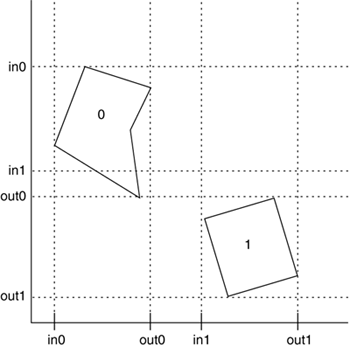# Mesh Versus Mesh (Sweep and Prune Approach)

The last algorithm we will discuss for collision detection is very well suited for environments consisting of many different objects. This kind of problem usually requires NxN tests (all objects can collide with all other objects), which are costly and must always be avoided. Sweep and prune allows us to focus only on potential collision candidates, because most object interactions will be discarded in an early, low CPU cost stage.

The key idea is to detect bounding box overlaps (see Figure 22.8). Two bounding boxes overlap if and only if their intervals overlap in all three directions, X, Y, and Z. So, we build three lists and insert these intervals into them. By analyzing the intervals, we can quickly detect which boxes overlap and perform a fine-grained test to actually compute the collision point.

##### Figure 22.8. A diagram showing how sweep and prune creates intervals in the different axes.Here is the full sweep and prune algorithm:

` Construct 3 lists, one for each dimension. For each object in the scene    project each 3-dimensional bounding box onto the x,y and z axes.    Store these projected values as ENTRANCE or EXIT in the lists End for Each list contains the end-points of the intervals corresponding to that dimension. Sort lists by coordinate value to determine which intervals overlap Scan the lists. Store overlaps in X,Y and Z in a separate array If an overlap occurs in three directions    // fine collision algorithm goes here end if `

In general, such a sort will take O(n log n) time. However, we can reduce this time if we keep the sorted lists from the previous frame, changing only the values of the interval endpoints. Because there will be relatively small movements between frames, the lists will be nearly sorted, so we can sort in expected O(n) time using Insertion Sort.Core Techniques and Algorithms in Game Programming2003
ISBN: N/A
EAN: N/A
Year: 2004
Pages: 261